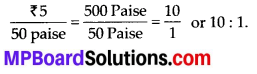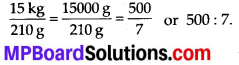# MP Board Class 7th Maths Solutions Chapter 8 Comparing Quantities Ex 8.1

## MP Board Class 7th Maths Solutions Chapter 8 Comparing Quantities Ex 8.1

Question 1.
Find the ratio of:
(a) ₹ 5 to 50paise
(b) 15 kg to 210 g
(c) 9 m to 27 cm
(d) 30 days to 36 hours
Solution:
(a) ₹ 5 to 50 paise
1 rupee = 100 paise
∴ 5 rupee = 500 paise
Hence, the required ratio is(b) 15 kg to 210 g
1kg = 1000g
15 kg = 15000 g
Hence, the required ratio is(c) 9 m to 27 cm
1m = 100 cm
9 m = 900 cm
Hence, the required ratio is(d) 30 days to 36 hours
1 day = 24 hours
30 days = 24 × 30 = 720 hrs
Hence, the required ratio isQuestion 2.
In a computer lab, there are 3 computers for every 6 students. How many computers will
be needed for 24 students?
Solution:
For 6 students, number of computers required = 3
For 1 student, number of computers required = $$\frac{3}{6}$$
For 24 students, number of computers required = $$24 \times \frac{3}{6}=12$$
Hence, 12 computers are required for 24 students.

Question 3.
Population of Rajasthan = 570 lakhs and population of UP = 1660 lakhs.
Area of Rajasthan = 3 lakhs km2 and Area of UP = 2 lakhs km2
(i) How many people are there per km2 in both these states ?
(ii) Which states is less populated?
Solution:
(i) Population of Rajasthan in 3 lakhs km2
area = 570 lakhs
Population of rajasthan in 1 km2 area
$$=\frac{570}{3}=190$$ lakhs
Population of U.P. in 2 km2 = 1660 lakhs
Population of U.P. in 1 km2 = $$\frac{1660}{2}$$
= 830 lakhs

(ii) It can be observed that population per km2 area is lesser for Rajasthan. Therefore, Rajasthan is less populated.